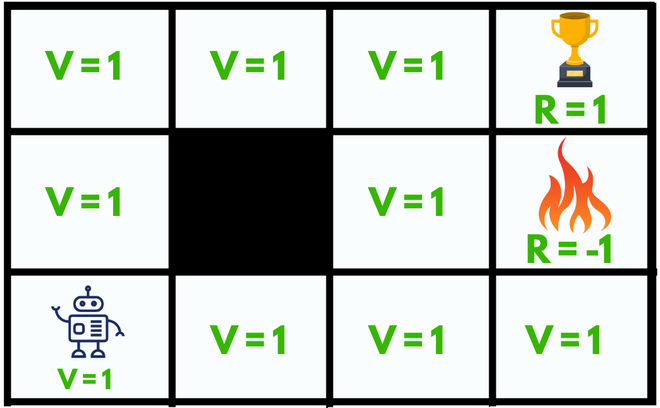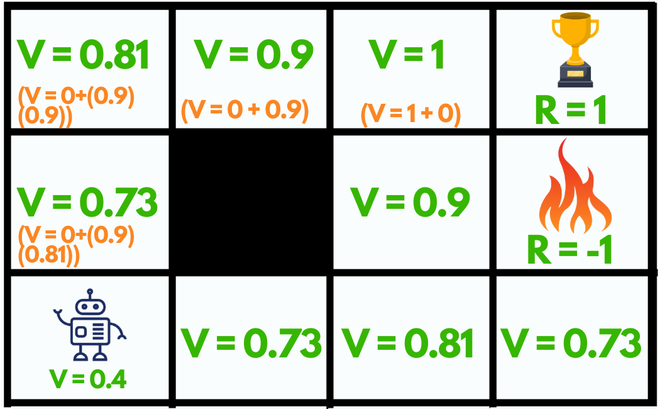Bellman Equation

• Difficulty Level : Hard
• Last Updated : 27 Sep, 2021

According to the Bellman Equation, long-term- reward in a given action is equal to the reward from the current action combined with the expected reward from the future actions taken at the following time. Let’s try to understand first.

Let’s take an example:

Here we have a maze which is our environment and the sole goal of our agent is to reach the trophy state (R = 1) or to get Good reward and to avoid the fire state because it will be a failure (R = -1) or will get Bad reward.Fig: Without Bellman Equation

What happens without Bellman Equation?

Initially, we will give our agent some time to explore the environment and let it figure out a path to the goal. As soon as it reaches its goal, it will back trace its steps back to its starting position and mark values of all the states which eventually leads towards the goal as V = 1.

The agent will face no problem until we change its starting position, as it will not be able to find a path towards the trophy state since the value of all the states is equal to 1. So, to solve this problem we should use Bellman Equation:

V(s)=maxa(R(s,a)+ γV(s’))

State(s): current state where the agent is in the environment

Next State(s’): After taking action(a) at state(s) the agent reaches s’

Value(V): Numeric representation of a state which helps the agent to find its path. V(s) here means the value of the state s.

Reward(R): treat which the agent gets after performing an action(a).

• R(s): reward for being in the state s
• R(s,a): reward for being in the state and performing an action a
• R(s,a,s’): reward for being in a state s, taking an action a and ending up in s’

e.g. Good reward can be +1, Bad reward can be -1, No reward can be 0.

Action(a): set of possible actions that can be taken by the agent in the state(s). e.g. (LEFT, RIGHT, UP, DOWN)

Discount factor(γ): determines how much the agent cares about rewards in the distant future relative to those in the immediate future. It has a value between 0 and 1. Lower value encourages short–term rewards while higher value promises long-term rewardFig: Using Bellman Equation

The max denotes the most optimum action among all the actions that the agent can take in a particular state which can lead to the reward after repeating this process every consecutive step.

For example:

• The state left to the fire state (V = 0.9) can go UP, DOWN, RIGHT but NOT LEFT because it’s a wall(not accessible). Among all these actions available the maximum value for that state is the UP action.
• The current starting state of our agent can choose any random action UP or RIGHT since both lead towards the reward with the same number of steps.

By using the Bellman equation our agent will calculate the value of every step except for the trophy and the fire state (V = 0), they cannot have values since they are the end of the maze.

So, after making such a plan our agent can easily accomplish its goal by just following the increasing values.

My Personal Notes arrow_drop_up